# Updated Post on How to Add LaTex to Your Posts

I know this is my third post today, but this is not a data post. It’s more of a PSA. If you want to add beautiful equations into your post (like I did here) then rather than generating a bunch of images and inserting them into the post, you can simply use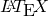.is the programming language for seamless integration of mathematical equations into manuscripts and is very nice. It does require a bit of knowledge of the language, but it is very straightforward and I have attached a PDF with the most common things that we would want to add and theirsyntax:

LaTeXSymbols

All we have to do to begin usingis type the command (latexpage) at the top of the page like so: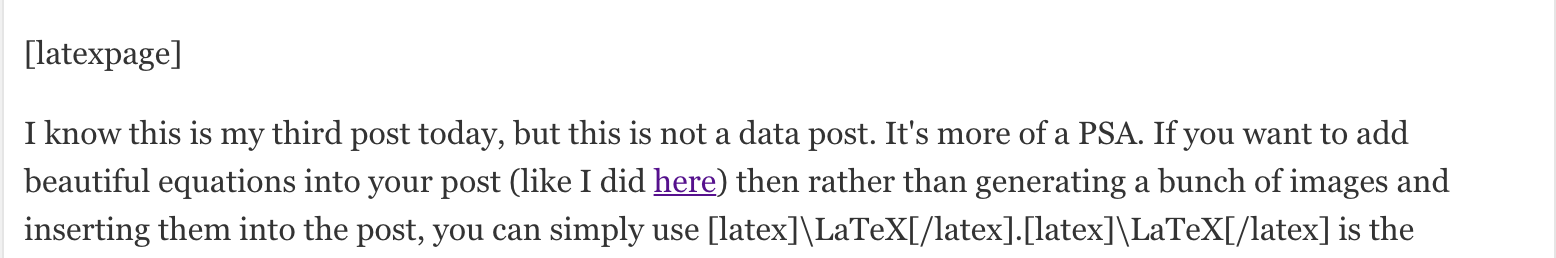Note, whenever you type this command and almost any other command that is in line with the text, you have to use SQUARE brackets, not parentheses. I am using parentheses simply because if I type square brackets you won’t be able to see the commands. For equations, you don’t need square brackets, which will you can see in my equation example below. This command will then allow you to type text like normal but when you want to add an equation, you simply have to use the begin{equation} and end{equation} commands. These are prefaced by the \ symbol like everything in(look at the reference document if you aren’t sure what I mean). Everything between the begin and end commands will be formatted as an equation and will even have their own equation number. I will demonstrate with the Hagen-Poiseuille equation:

(1)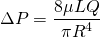which would look something like this in the edit post window: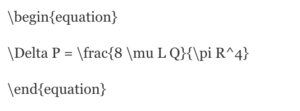The only other thing that you need to consider is if you want to add something in line with the text, like I am with the, then you have to do either (latex) your_arg_here (/latex) or (math) your_arg_here (/math) except with square brackets. This would give you something like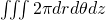. Note for these the slash symbol is /, not \. Also, when you have things that you want paired, then put a space between them. While not totally necessary for text, when you are doing something like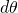or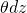, you don’t want to do d\theta or \thetadz, both of which are not recognized by. It is best to just get in the habit of either typing d \theta or \theta d z. Here is this paragraph as an image so that you can see what I am doing: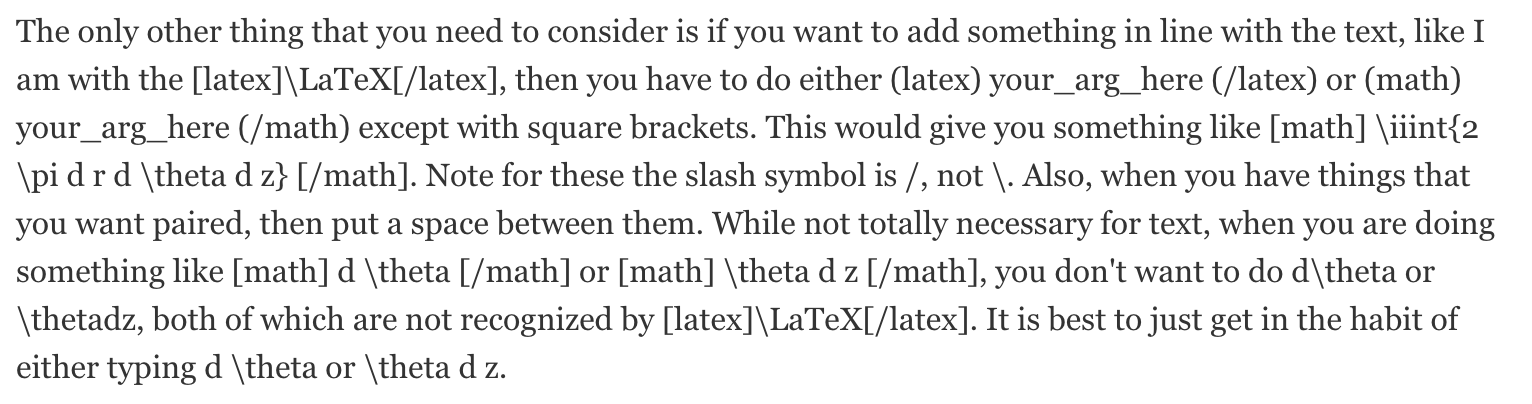This should suffice for any basicneeds that you have and if you wish for more knowledge, then I would recommend going here. Otherwise, I’d be more than happy to help if you have questions, so you can feel free to email me.